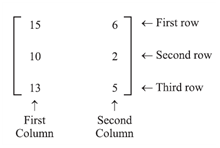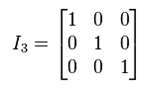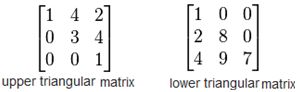# CBSE Class 12th Matrices- Various Types of Matrices

In this video we will learn about the topics Matrices, Various types of Matrices ,Representation of Matrices and Order of Matrices.

Jan 29, 2015 18:46 IST

In this video we will learn about the topics Matrices, Various types of Matrices ,Representation of Matrices and Order of Matrices

A set of mn numbers (real or imaginary) arranged in the form of rectangular array of m rows and n columns is called an m x n matrix.

Let’s have a look on the example given below:In the above example, there are 3 rows and 2 columns. So, m =3,  n= 2 and we can call it as 3 x 2 matrix

Summary:

• A set of mn numbers (real or imaginary) arranged in the form of rectangular array of m rows and n columns is called an m x n matrix.
• [aij] m × 1 is a column matrix.
• [aij] 1 × n is a column matrix
• In a matrix, A = [aij] m × n , where m represents the number of rows and n represents the number of columns.
• m x n is called the order of matrix
• if m≠ n, then the matrix is called as a rectangular matrix
• if m = n, then the matrix is called as a square matrix
• Identity matrix or unit matrix of size n is the n × n square matrix with ones on the main diagonal and zeros elsewhere.• A square matrix is called upper triangular if all the entries above the main diagonal are zero
• A square matrix is called lower triangular if all the entries below the main diagonal are zero• Zero or Null matrix : It can be a rectangular matrix or a square matrix
• All the elements of a null matrix are zero